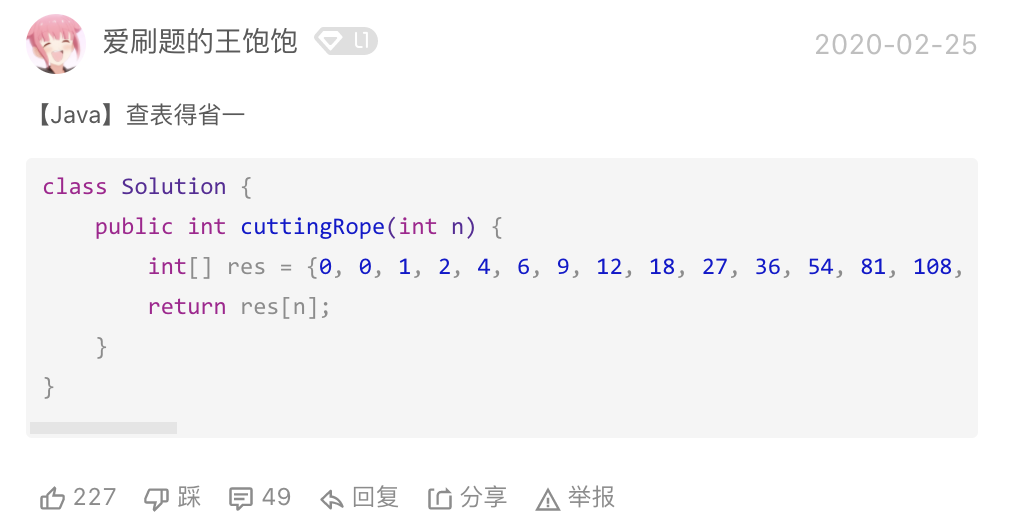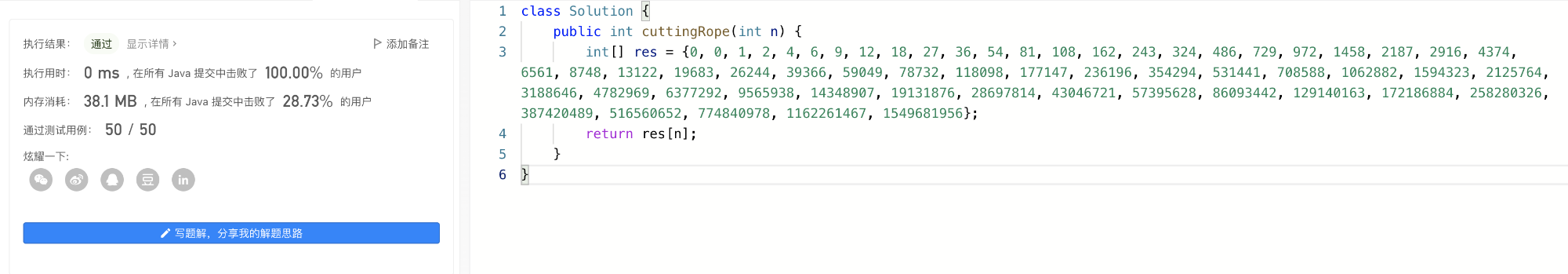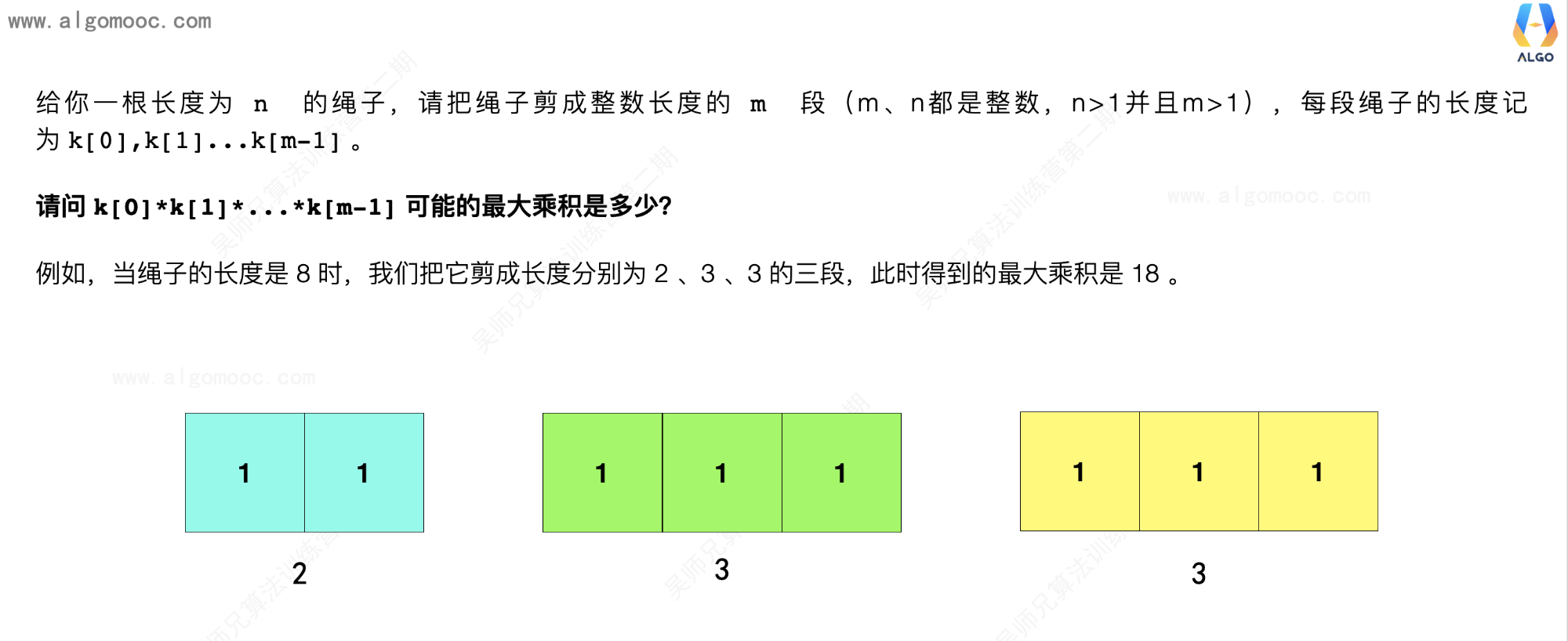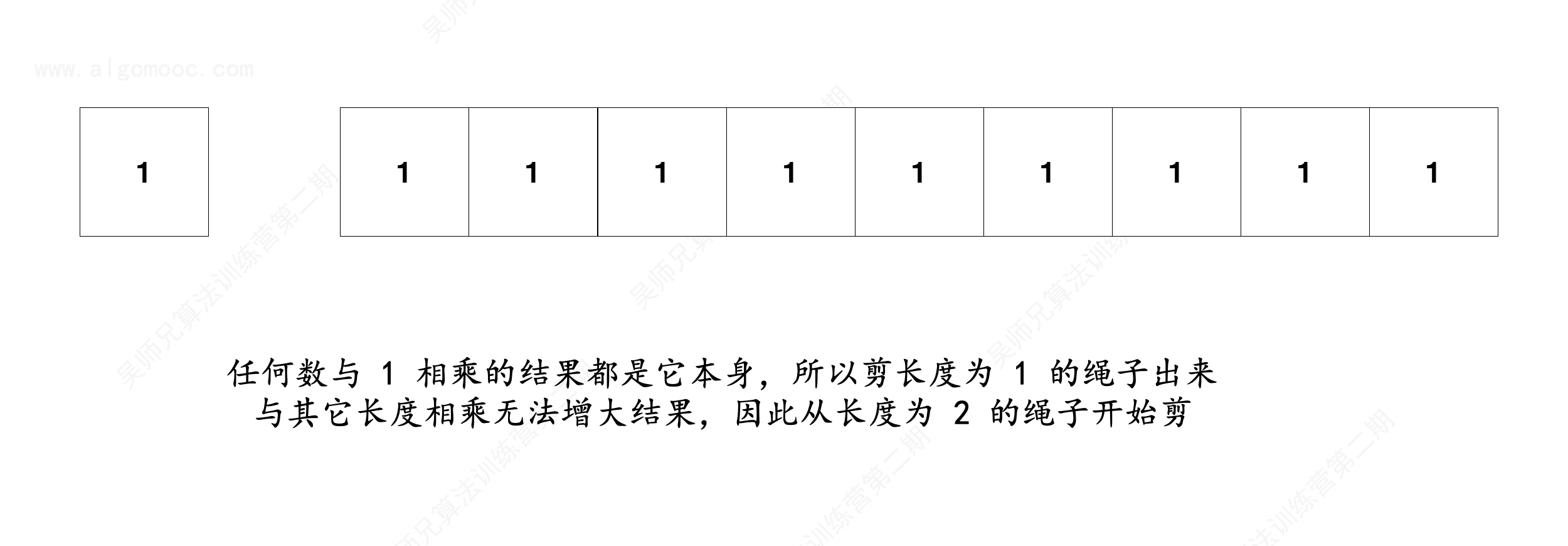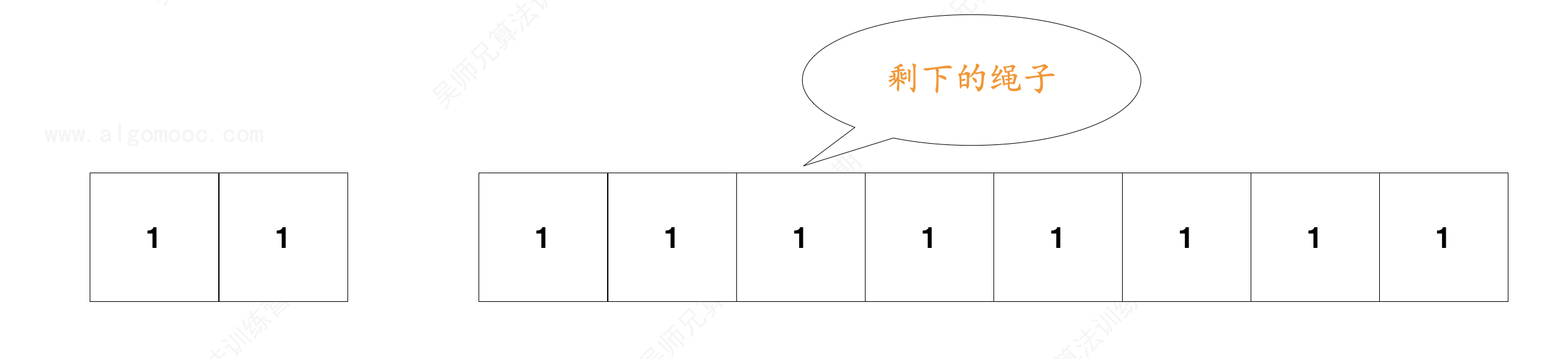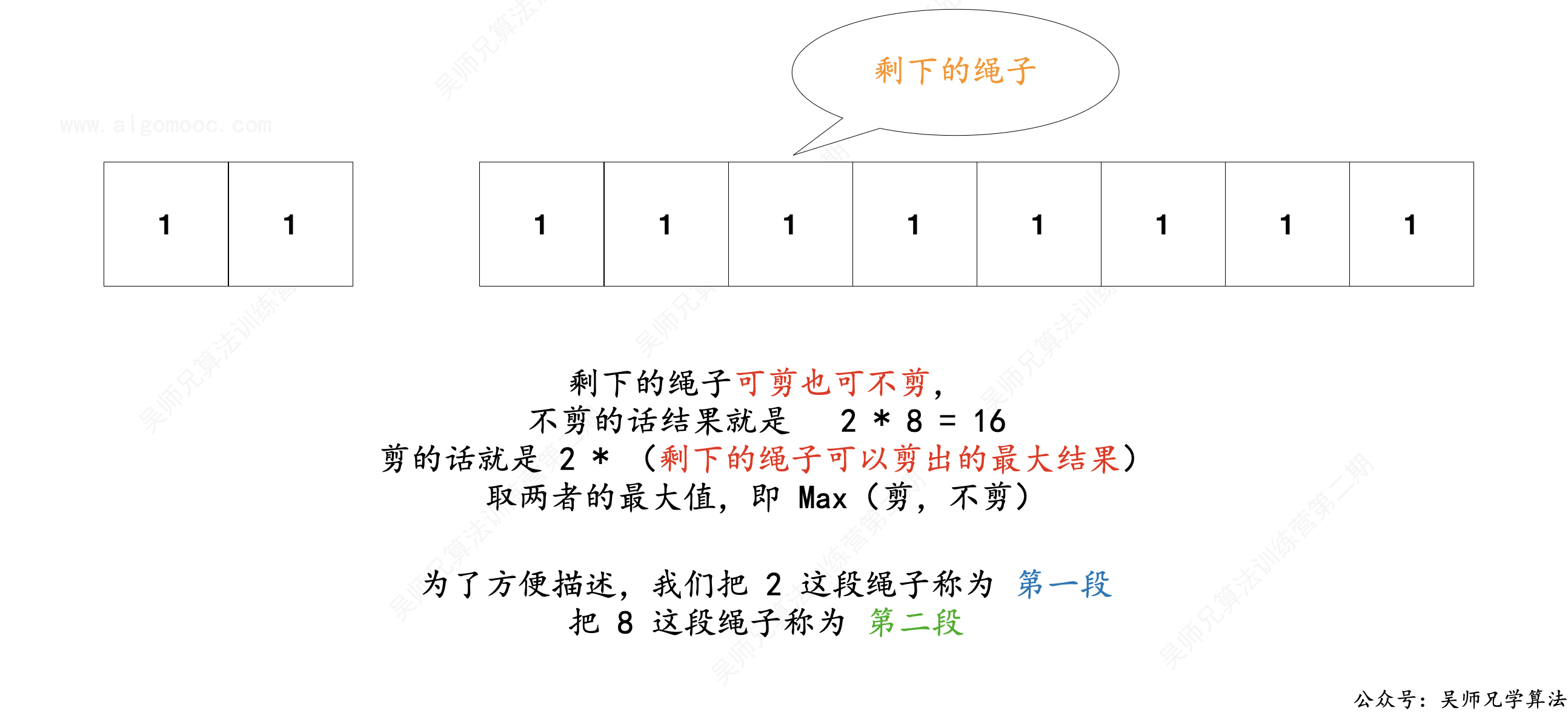• 不剪，乘积结果为  2 * 8 = 16
• 剪，怎么剪？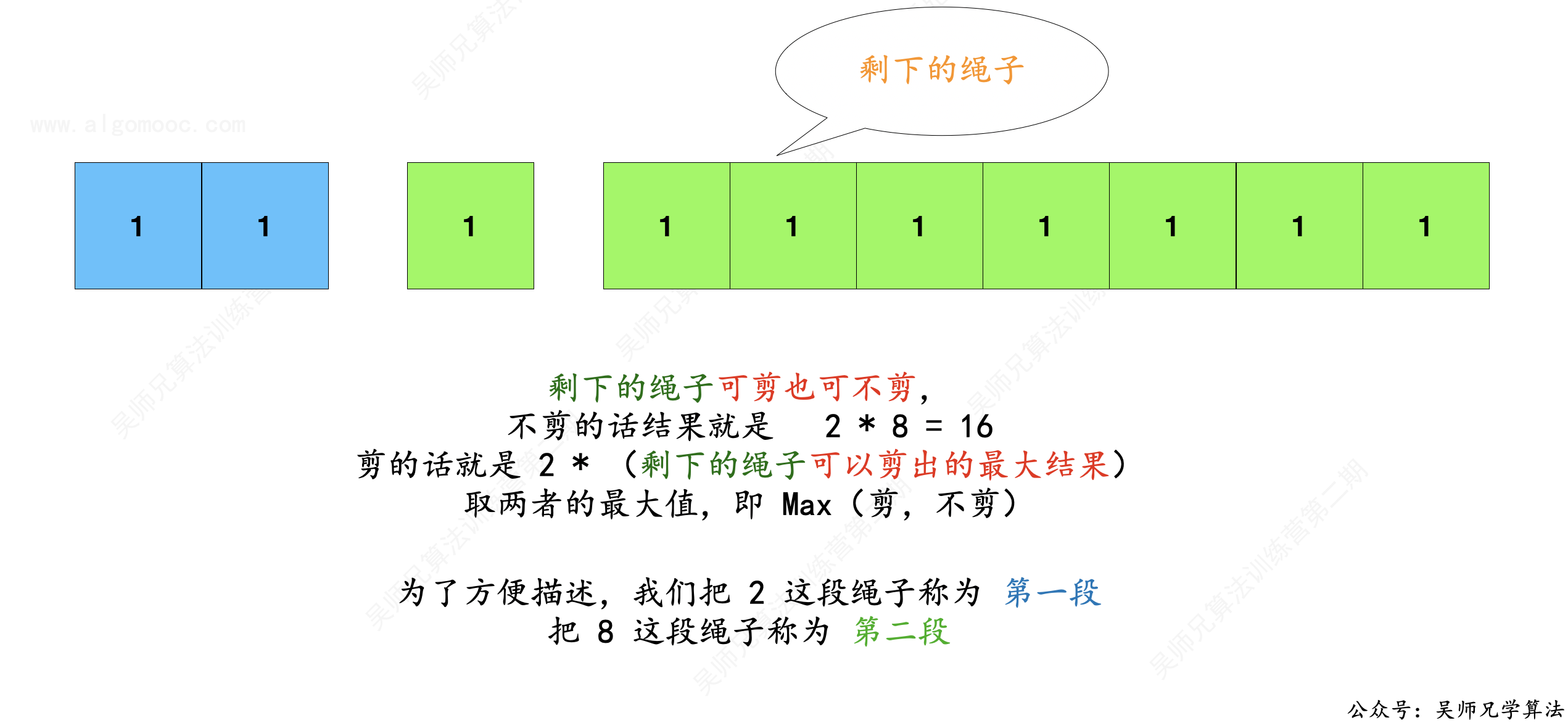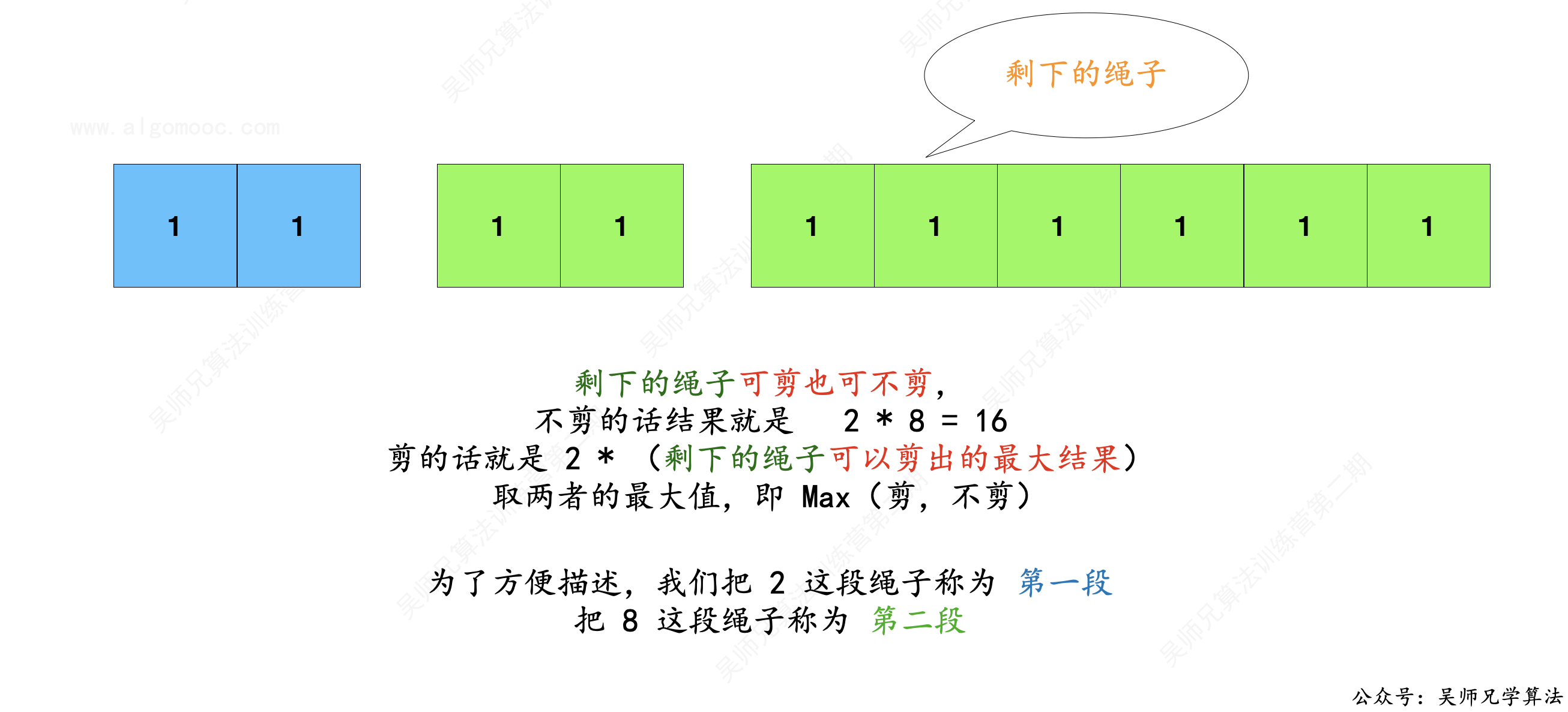// 登录 AlgoMooc 官网获取更多算法图解
// https://www.algomooc.com
// 作者：程序员吴师兄
// 代码有看不懂的地方一定要私聊咨询吴师兄呀
class Solution {
public int cuttingRope(int n) {
// 长度为 1 的绳子没法剪了
if ( n <= 1 ) return 1;
// 用一个名为 dp 的数组来记录从 0 到 n 长度的绳子剪掉后的最大乘积
// 默认都是 0
int[] dp = new int[n + 1];
// 由于绳子必须被剪，所以长度为 2 的绳子只有剪成 1 和 1 的两段，乘积结果为 1
dp = 1;
// 长度大于等于 3 的绳子才开始进入我们的讨论范围
// 从长度为 3 的绳子开始考虑，讨论它的最大乘积是多少，并不断的延伸绳子的长度
for(int i = 3; i < n + 1; i++){
// 对于长度为 i 的绳子，它可以分为两个区间 j 和 i - j
// j 的范围由 2 开始，因为剪长度为 1 的绳子无法扩大乘积的值
// j 的范围可以延伸至 i - 1
for(int j = 2; j < i; j++){
// 不剪总长度乘积为  j * （ i - j）
// 剪的话长度乘积为  j * dp[ i - j ]
// 取两者的最大值，即  Max ( j * ( i - j) , j * dp[ i - j] )
// 那么此时 dp[i] 的值取 i 不剪的值（ dp[i]) 和剪的值 Math.max(j * (i - j), j * dp[i - j]) 这两者的最大值
// 比如一开始 i = 3 ， j = 2
// dp = Math.max( 0 ,Math.max ( 2 * 1, 2 * dp)
//       = Math.max( 0 ,Math.max ( 2, 2))
//       = 2
dp[i] = Math.max(dp[i], Math.max(j * (i - j), j * dp[i - j]));
}
}
return dp[n];
}
}